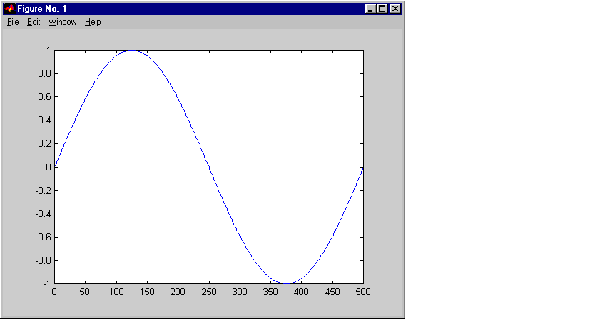External InterfacesCalling Functions from Fortran MEX-Files

It's possible to call MATLAB functions, operators, M-files, and even other MEX-files from within your Fortran source code by using the API function `mexCallMATLAB`. This example creates an `mxArray`, passes various pointers to a subfunction to acquire data, and calls `mexCallMATLAB` to calculate the sine function and plot the results.

• ```C==============================================================
=
C     sincall.f
C
C     Example for illustrating how to use mexCallMATLAB.
C
C     Creates an mxArray and passes its associated pointers (in
C     this demo, only pointer to its real part, pointer to number
C      of rows, pointer to number of columns) to subfunction fill()
C      to get data filled up, then calls mexCallMATLAB to calculate
C     sin function and plot the result.
C
C     NOTE: The subfunction fill() is in the file called fill.f.
C
C     This is a MEX-file for MATLAB.
C     Copyright (c) 1984-2000 The MathWorks, Inc.
C     \$Revision: 1.10 \$
C ==============================================================

C     The gateway routine
subroutine mexFunction(nlhs, plhs, nrhs, prhs)

integer mxGetPr, mxCreateDoubleMatrix
integer plhs(*), prhs(*)
integer lhs(1), rhs(1)
integer nlhs, nrhs
integer m, n, max

C     initializition
m = 1
n = 1
max = 1000

rhs(1) = mxCreateDoubleMatrix(max, 1, 0)

C     Pass the pointer and variable and let fill() fill up data.
call fill(%val(mxGetPr(rhs(1))), m, n, max)
call mxSetM(rhs(1), m)
call mxSetN(rhs(1), n)

call mexCallMATLAB(1, lhs, 1, rhs, 'sin')
call mexCallMATLAB(0, NULL, 1, lhs, 'plot')

C     Clean up the unfreed memory after calling mexCallMATLAB.
call mxDestroyArray(rhs(1))
call mxDestroyArray(lhs(1))

return
end
```

This is the subroutine that `sincall` calls to fill the mxArray with data.

• ```C==============================================================
=
C     fill.f
C     This is the subfunction called by sincall that fills the
C     mxArray with data. Your version of fill can load your data
C     however you would like.
C
C     This is a MEX-file for MATLAB.
C     Copyright (c) 1984-2000 The MathWorks, Inc.
C     \$Revision: 1.3 \$
C==============================================================
=

C     Subroutine for filling up data.
subroutine fill(pr, m, n, max)
real*8  pr(*)
integer i, m, n, max

m = max/2
n = 1
do 10 i=1,m
10      pr(i) = i*(4*3.1415926/max)

return
end
```

It is possible to use `mexCallMATLAB` (or any other API routine) from within your computational Fortran subroutine. Note that you can only call most MATLAB functions with double-precision data. M-functions that perform computations, like `eig`, will not work correctly with data that is not double precision.

Running this example

• ```sincall
```

displays the resultsNote    It is possible to generate an object of type `mxUNKNOWN_CLASS` using `mexCallMATLAB`. See the example below.

The following example creates an M-file that returns two variables but only assigns one of them a value.

• ```function [a,b]=foo[c]
a=2*c;
```

MATLAB displays the following warning message.

• ```Warning: One or more output arguments not assigned during call to
'foo'.
```

If you then call `foo` using `mexCallMATLAB`, the unassigned output variable will now be of type `mxUNKNOWN_CLASS`.Handling Sparse Matrices Advanced Topics© 1994-2005 The MathWorks, Inc.